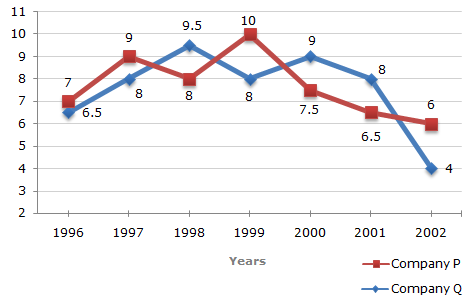# Data Interpretation - Line Charts - Discussion

### Discussion :: Line Charts - Line Chart 8 (Q.No.5)

Two different finance companies declare fixed annual rate of interest on the amounts invested with them by investors. The rate of interest offered by these companies may differ from year to year depending on the variation in the economy of the country and the banks rate of interest. The annual rate of interest offered by the two Companies P and Q over the years are shown by the line graph provided below.

Annual Rate of Interest Offered by Two Finance Companies Over the Years.5.

An investor invested Rs. 5 lakhs in Company Q in 1996. After one year, the entire amount along with the interest was transferred as investment to Company P in 1997 for one year. What amount will be received from Company P, by the investor?

 [A]. Rs. 5,94,550 [B]. Rs. 5,80,425 [C]. Rs. 5,77,800 [D]. Rs. 5,77,500

Explanation:

Amount received from Company Q after one year on investment of Rs. 5 lakhs in the year 1996

= Rs. [5 + (6.5% of 5)] lakhs

= Rs. 5.325 lakhs.

Amount received from Company P after one year on investment of Rs. 5.325 lakhs in the year 1997

= Rs. [5.325 + (9% of 5.325)] lakhs

= Rs. 5.80425 lakhs

= Rs. 5,80,425.

 Sunny said: (May 28, 2014) "What amount will be received from Company P, by the investor?" Should it not be TOTAL amount I subtracted out the interest as this is the "amount" actually received "from Company P".

 Om Prakash Mahto said: (May 31, 2014) Understanding the question is the trick to solve this type of question in a faster way. This question can be done in a simpler way if the student right away see that is nothing but a question of compound interest for 2 years. The interest are 605 and 9 for the amount 5 lacks. So total interest after 2 years is : 6. 5+9+ ( (6. 5*9) /100). Ie; by using formula x+y+xy/100. So interest is 16. 085%. And interest is 500000*16. 085% = 80425. Now simple 500000 + 80425 is the answer or just you can see the option after calculating the interest.

 Srinivas said: (May 11, 2017) In 1996, 5,00,0000 invested for 6.5%interest. 5,00,000 * 6.5/100 = 32,500. Received = 5,00,000 + 32,500 = 5,32,5000. Then invested for one more year in another company for 9% interest. So, 5,32,500 * 9/100 = 47,925. Principle amount 5,32,500+ interest amount 47,925. Answer is= 5,80,425.